# fx-CP400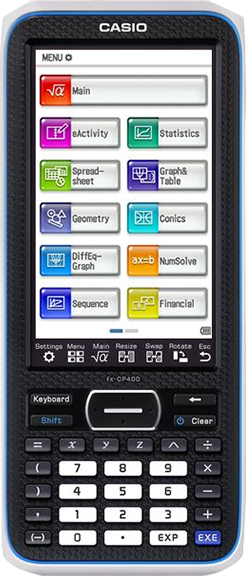# fx-CP400

## Easy-to-operate, CAS(Computer Algebra System)features provide visual support for mathematical exploration.

fx-CP400 has intuitive operability and exploring features, ideal for mathematics learning.

OS Update Information

• Calculation capability improvement.

# Features

## 1 Easy and Intuitive operability

Intuitive feature makes it easy to use the features of fx-CP400.

This feature makes the fx-CP400 ideal for mathematics learning.

•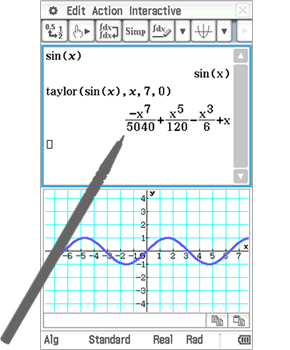## 1Touch panel

The fx-CP400 offers intuitive operation through use of the stylus on the touch panel.

•## 2Pinch-in and Pinch-out

Intuitive pinch-in / pinch-out control allows you to easily adjust a graph to the exact display size that you desire as zoom in or zoom out.

•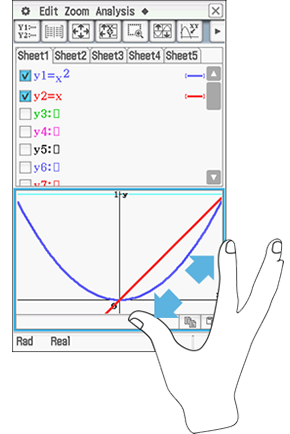•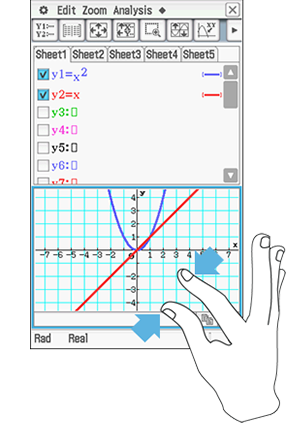## 3Slider function

By slider function to the Graph / Geometry the control to freely change values allows you to learn their relationship with other values by freely changing shapes

•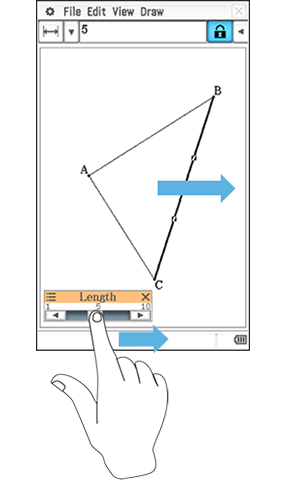## 4Drag and drop

Mathematical formulas can be graphically displayed without any complicated key operations - simply drag and drop the formula into the graph area!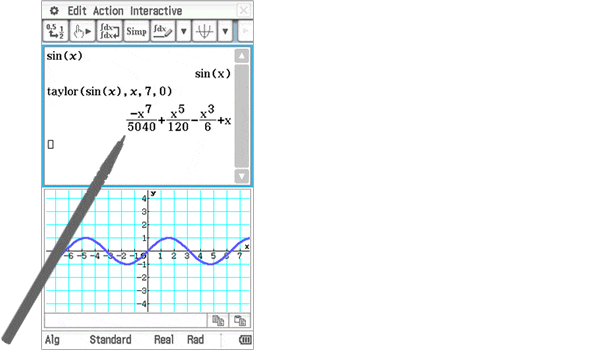## 2 Essential functions for learning all in one

CAS Graphing calculators have a variety of functions covering from primary to higher education. Students can cultivate mathematical thinking through Graph functions operated by themselves.

•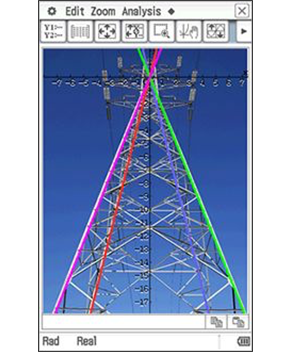(C) pn_photo - Fotolia.com

•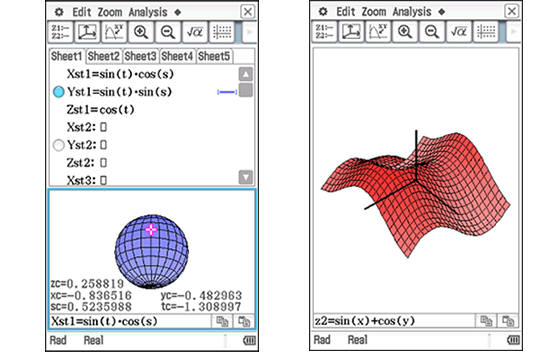3D Graph function3D Graph function

## 1Calculation using CAS

CAS(Computer Algebra System) is a software program facilitating symbolic mathematics.

•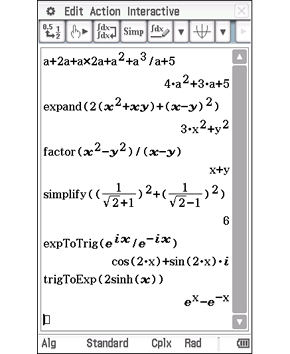Expand, Factor, Simplify calculations

•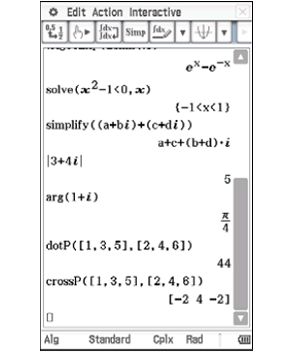Solve, Complex, Vector calculations

•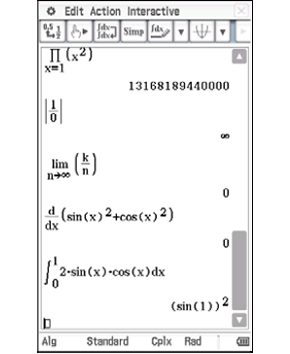Limit, Differentiation, Integration calculations

•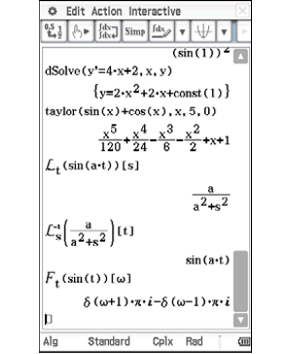Differential equation, Taylor polynomial, Laplace transform calculations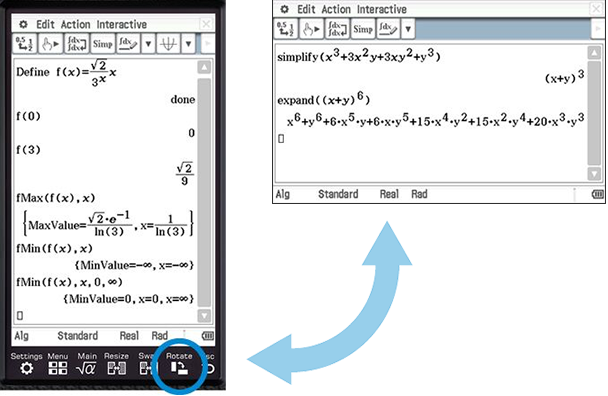Upright? Horizontal? Both!

### Interactive Differential Calculus

Visual, intuitive operations make it possible to learn the concept of differentials which is often hard to understand for students.

•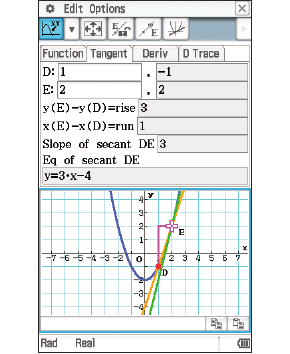Function and tangents

•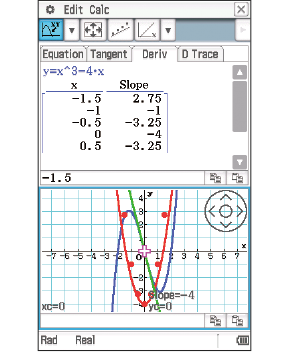Plot coordinates of the slope of a tangent and predict the function

•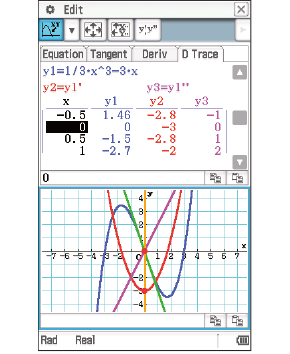Function and its first derivative formula

You can learn differential coefficients and derivative formulas by exploring this function.

### Differential Equation Graph

The solution set of differential equations can be represented as graphs in vector fields, and solution curves can be drawn by providing initial conditions of the equations.

•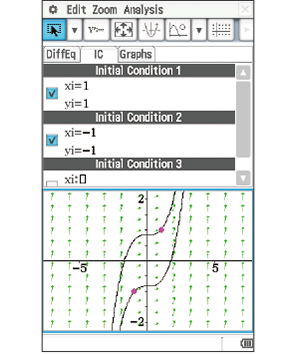Slope field and solution curves for the initial conditions

## 2Graphing function

### Graph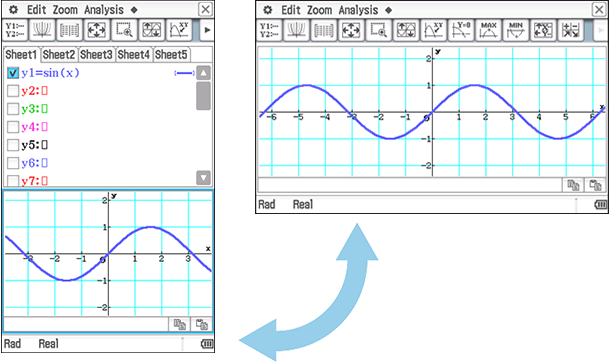Upright view - Horizontal view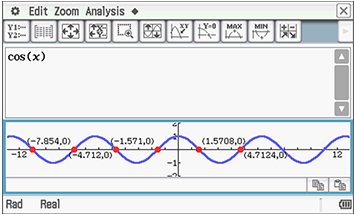Formula and Graph at the same time

### Graph

•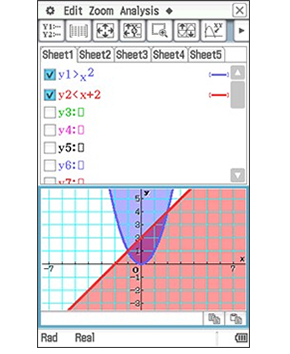Inequality Graph

•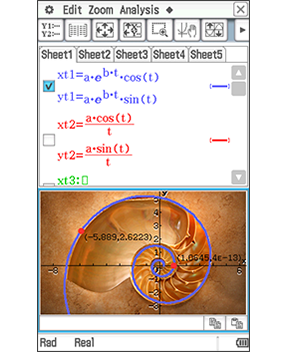Parametric Graph

### 3D Graph

Use of the 3-dimensional graph drawing function allows for the depiction of more complicated graphs. Supports the study of topics such as “spheres” and “planes,” which can be difficult to understand from just a textbook or class notes.

•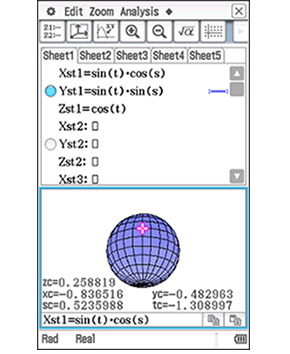•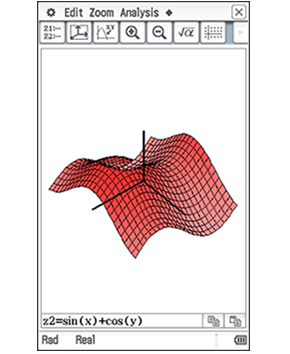## Example

### Explore in a variety of ways.

•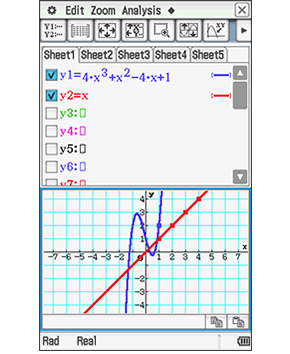Equation and Graph

••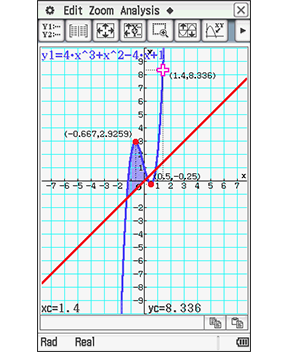Graph

••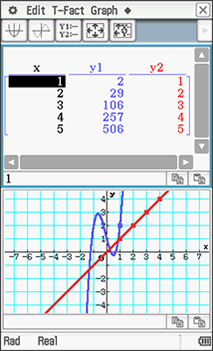Graph and Table

•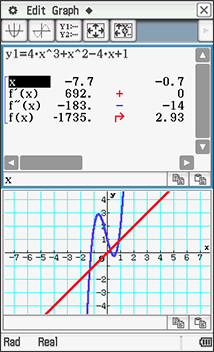Graph and Summary TableGraph and Summary Table

## 3Other function

### Statistics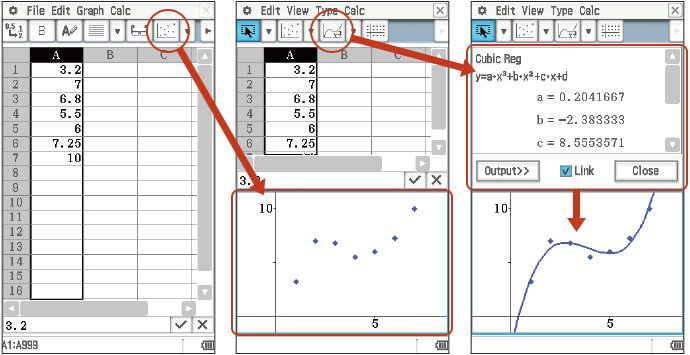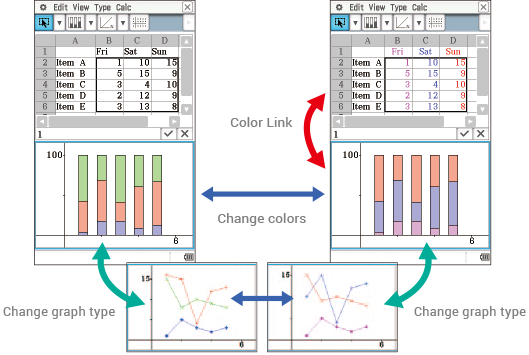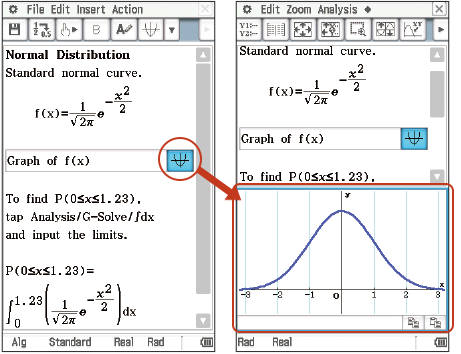Statistics using eActivity function

### Geometry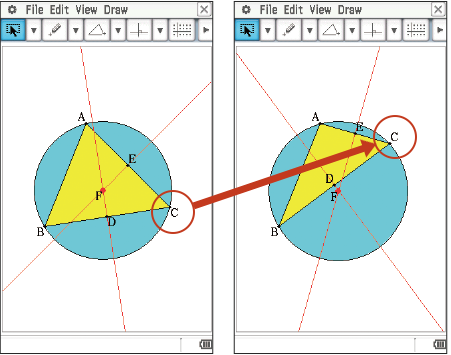Geometric graphing

•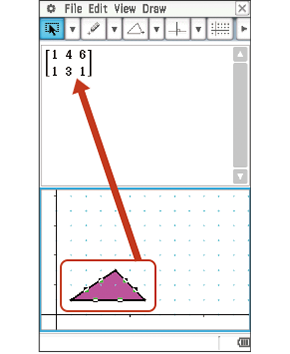•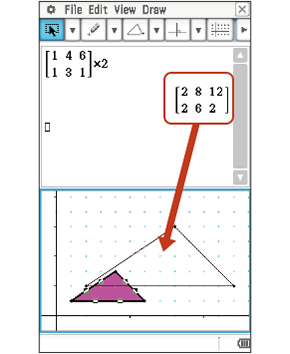Geometric graphing using drag and drop

•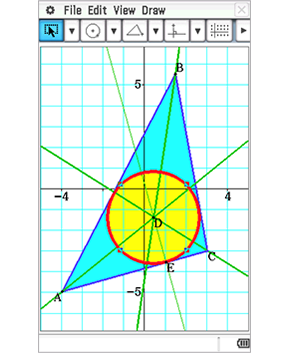Triangle and circle

## Example

Explore the relationship between formula and Geometry

•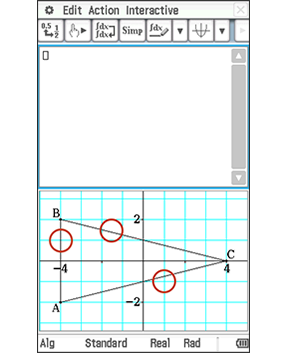Triangle

•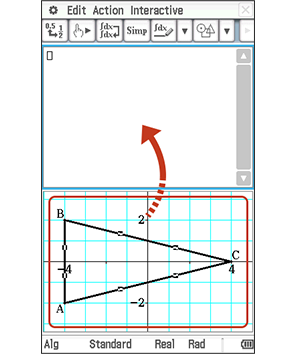Drag and drop

•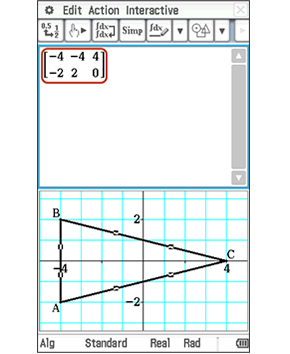Formula

Explore the relationship between Length, Angle and Area of triangle.

• “Length”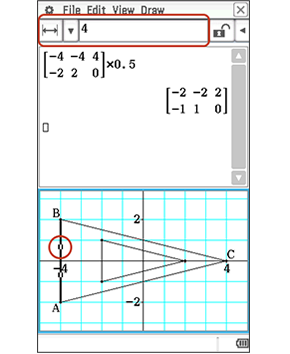Tap AB

• “Angle”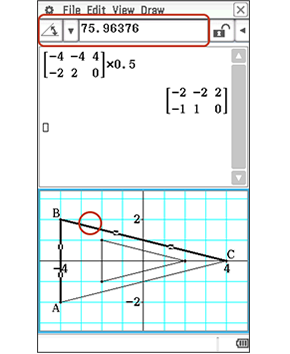Tap BC

• “Area”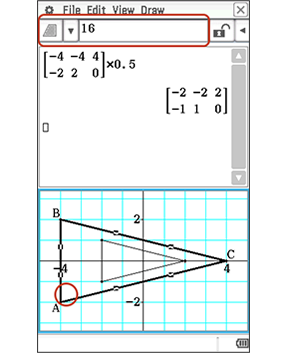Tap AC

• “Smaller triangle”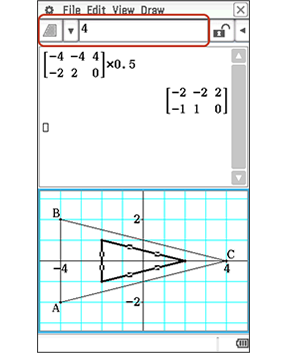Tap in any white space

## 3 Extensive and advanced features

Data-logger can be used with graphing calculators and is effective for both mathematics education and science and technology education.

•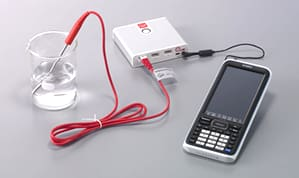Science and Technology Education

## 1E-CON3

E-CON is an application used to operate a data logger, a device used to collect data. E-CON makes it easy to collect data for use in classroom science and technology lessons.

•This feature is useful in science experiments

## 2Applied studying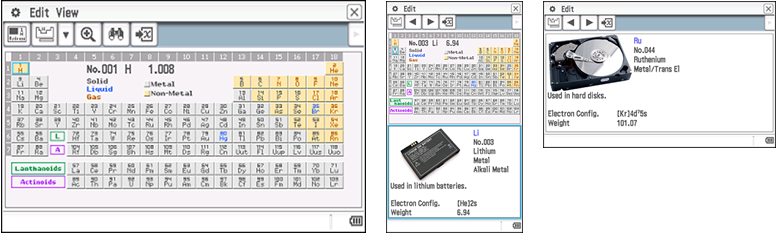Periodic Table

•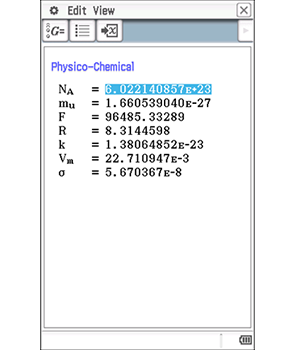Fundamental Physical Constants

•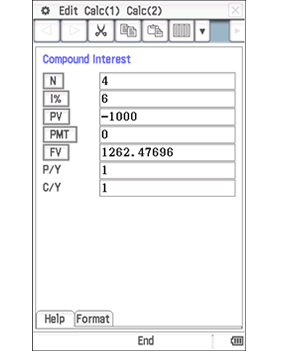Financial

## 3Exam Mode

This mode allows you to quickly prepare your calculator for exams. This mode restricts access to memory, programs, functions and applications, so that these features would not be available during exams.

•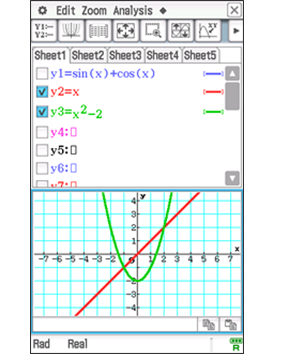# Teacher's Voice## School

Math and Science Division Chair at Lyons Township High School
LaGrange Illinois (USA)

Ismael Zamora

## Comment

The invention of the graphing calculator forever changed the face of mathematics education. I feel that the Casio fx-CP400 has once again changed how we teach mathematics. The ability to interact with equations and graphs on a single screen is revolutionary. I can now focus on the connections between concepts. The fx-CP400 allows me to explore connections between geometry, statistics and data analysis through the use of data collections. It allows my students to make conjectures and model the mathematics that is right in front of them. The ability to graph in 3D allows my students to see the world in a whole new way and allows them to ask and answer questions I have never been able to ask them before.